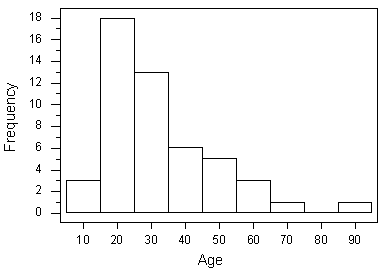# STAT 200 Examination Check point 02

## Question 1

The distribution of the amount of money spent by students for textbooks in a semester is approximately normal in shape with a mean of \$235 and a standard deviation of \$20. According to the standard deviation rule, how much did almost all (99.7%) of the students spend on textbooks in a semester?

## Question 2

The histogram below displays the distribution of 50 ages at death due to trauma (unnatural accidents and homicides) that were observed in a certain hospital during a week.Which of the following are the appropriate numerical measures to describe the center and spread of the above distribution?

## Question 3

A student survey was conducted at a major university, and data were collected from a random sample of 750 undergraduate students. One variable that was recorded for each student was the student's answer to the question: “What region of the country did you live in just prior to enrolling in this university? Northeast/Southeast/Northwest/Southwest/Midwest/Outside the U.S.”

These data would be best displayed using which of the following?

The next four questions relate to the same boxplot graph, shown below.

## Question 4

The boxplots below show the real estate values of single-family homes in two neighboring cities (in thousands of dollars).Which city has more households?

## Question 5

The boxplots below show the real estate values of single-family homes in two neighboring cities (in thousands of dollars).Which city has greater variability in real estate values?

## Question 6

The boxplots below show the real estate values of single-family homes in two neighboring cities (in thousands of dollars).Which city has the greater percentage of households with real estate values above \$85,000?

## Question 7

The boxplots below show the real estate values of single-family homes in two neighboring cities (in thousands of dollars).Which city has a greater percentage of homes with real estate values between \$55,000 and \$85,000?

## Question 8

What determines which numerical measures of center and spread are appropriate for describing a given distribution of a quantitative variable?
• Posted: 4 years ago
A+ SOLUTION

Purchase the answer to view itSave time and money!
Our teachers already did such homework, use it as a reference!